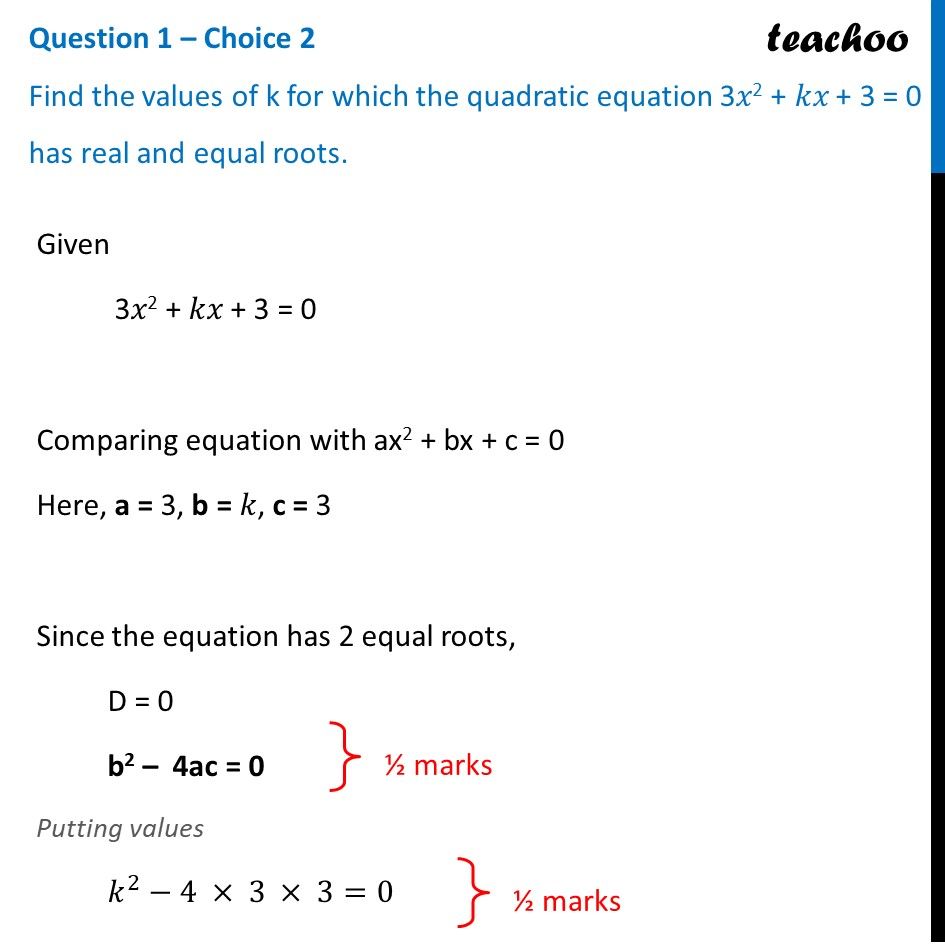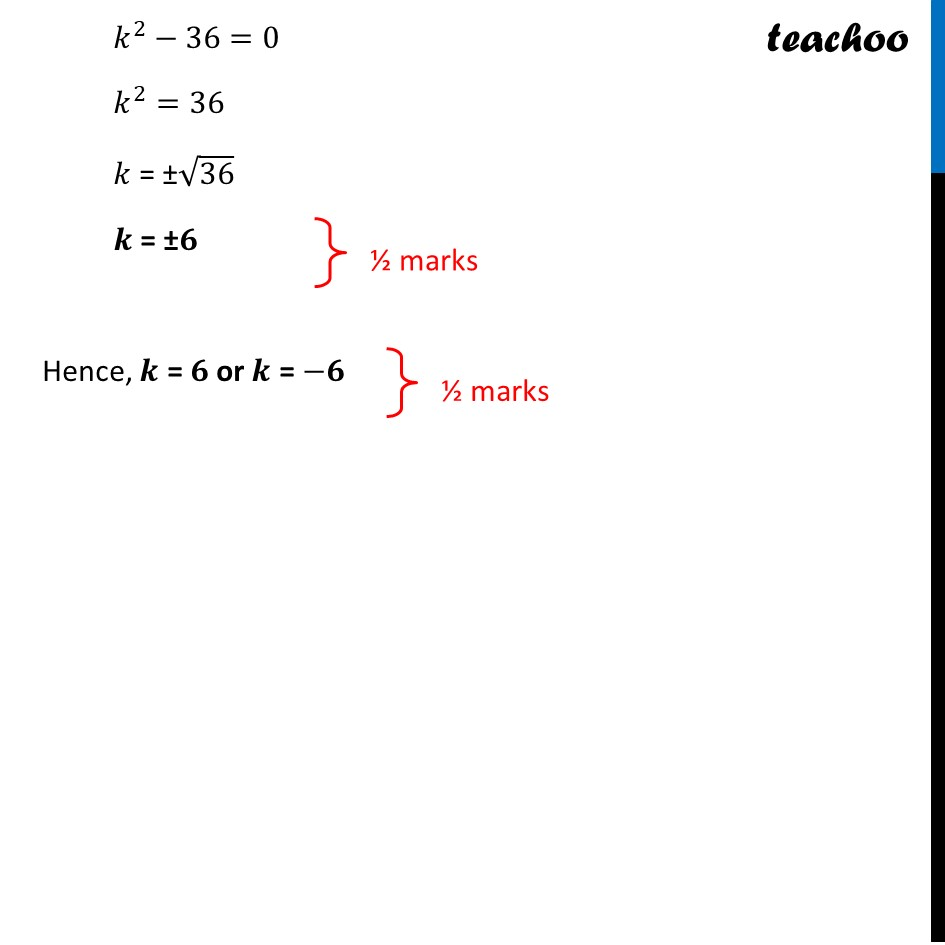CBSE Class 10 Sample Paper for 2022 Boards - Maths Basic [Term 2]

Class 10
Solutions of Sample Papers for Class 10 Boards

## Find the values of k for which the quadratic equation 3x 2 =  kx + 3 = 0 has real and equal roots.

This question is similar to Ex 4.4, 2 (i) Chapter 4 Class 10 - Quadratic EquationsLearn in your speed, with individual attention - Teachoo Maths 1-on-1 Class

### Transcript

Question 1 – Choice 2 Find the values of k for which the quadratic equation 3𝑥2 + 𝑘𝑥 + 3 = 0 has real and equal roots. Given 3𝑥2 + 𝑘𝑥 + 3 = 0 Comparing equation with ax2 + bx + c = 0 Here, a = 3, b = 𝑘, c = 3 Since the equation has 2 equal roots, D = 0 b2 – 4ac = 0 Putting values 𝑘^2−4 × 3 × 3=0 𝑘^2−36=0 𝑘^2=36 𝑘 = ±√36 𝒌 = ±𝟔 Hence, 𝒌 = 𝟔 or 𝒌 = −𝟔